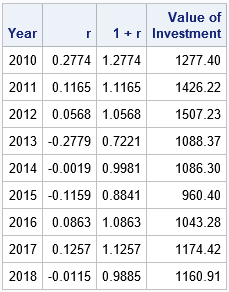There are several different kinds of means. They all try to find an average value from among a set of numbers. Although the most popular mean is the arithmetic mean, the geometric mean can be useful for problems in statistics, finance, and biology. A common application of the geometric mean is to find an average growth rate for an asset or for a population.

### What is the geometric mean?

The geometric mean of n nonnegative numbers is the n_th root of the product of the numbers:
GM = (x1 * x2 * ... * xn)1/n = (Π xi)1/n
When the numbers are all positive, the geometric mean is equivalent to computing the arithmetic mean of the log-transformed data and then using the exponential function to back-transform the result: GM = exp( (1/n) Σ log(xi) ).

### Physical interpretation of the geometric mean

The geometric mean has a useful interpretation in terms of the volume of an n-dimensional rectangular solid. If GM is the geometric mean of n positive numbers, then GM is the length of the side of an n-dimensional cube that has the same volume as the rectangular solid with side lengths x1, x2, ..., xn. For example, a rectangular solid with sides 1.5, 2, and 3 has a volume V = 9. The geometric mean of those three numbers are ((1.5)(2)(3))1/3 ≈ 2.08. The volume of a cube with sides of length 2.08 is 9.

This interpretation in terms of volume is analogous to interpreting the arithmetic mean in terms of length. Namely, if you have n line segments of lengths x1, x2, ..., xn, then the total length of the segments is the same as the length of n copies of a segment of length AM, where AM is the arithmetic mean.

### What is the geometric mean good for?

The geometric mean can be used to estimate the "center" of any set of positive numbers but is frequently used to estimate an average value in problems that deal with growth rates or ratios. For example, the geometric mean is an average growth rate for an asset or for a population. The following example uses the language of finance, although you can replace "initial investment" by "initial population" if you are interested in population growth

In precalculus, growth rates are introduced in terms of the growth of an initial investment amount (the principle) compounded yearly at a fixed interest rate, r. After n years, the principle (P) is worth
An = P(1 + r)n
The quantity 1 + r sometimes confuses students. It appears because when you add the principle (P) and the interest (Pr), you get P(1 + r).

In precalculus, the interest rate is assumed to be a constant, which is fine for fixed-rate investments like bank CDs. However, many investments have a growth rate that is not fixed but varies from year to year. If the growth rate of the investment is r1 during the first year, r2 during the second year, and rn during the n_th year, then after n years the investment is worth
An = P(1 + r1)(1 + r2)...(1 + rn)
= P (Π xi), where xi = 1 - ri.

What is the average growth rate for the investment? One interpretation of the average growth rate is the fixed rate that would give the same return after n years. That hypothetical fixed-rate growth is found by using the geometric mean of the values x1, x2, ..., xn. That is, if GM is the geometric mean of the xi, the value
An = P*GMn,
which assumes a fixed interest rate, is exactly the same as for the varying-rate computation.

### An example of the geometric mean: The growth rate of gold

Let's apply the ideas in the preceding section. Suppose that you bought \$1000 of gold on Jan 1, 2010. The following table gives the yearly rate of return for gold during the years 2010–2018, along with the value of the \$1000 investment at the end of each year.According to the table, the value of the investment after 9 years is \$1160.91, which represents a total return of about 16%. What is the fixed-rate that would give the same return after 9 years when compounded annually? That is found by computing the geometric mean of the numbers in the third column:
GM = (1.2774 * 1.1165 * ... * 0.9885)1/9 = 1.01672
In other words, the investment in gold yielded the same return as a fixed-rate bank CD at 1.672% that is compounded yearly for 9 years. The end-of-year values for both investments are shown in the following graph.### The geometric mean in statistics

The geometric mean arises naturally in situations in which quantities are multiplied together. This happens so often that there is a probability distribution, called the lognormal distribution, that models this situation. if Z has a normal distribution, then you can obtain a lognormal distribution by applying the exponential transformation: X = exp(Z) is lognormal. The Wikipedia article for the lognormal distribution states, "The lognormal distribution is important in the description of natural phenomena... because many natural growth processes are driven by the accumulation of many small percentage changes." For lognormal data, the geometric mean is often more useful than the arithmetic mean. In my next blog post, I will show how to compute the geometric mean and other associated statistics in SAS.

The post What is a geometric mean? appeared first on The DO Loop.